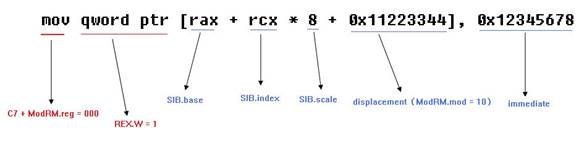# 6. SIB 补充寻址

### 上一页 返回目录 下一页

SIB 是对 ModRM 寻址的一个补充：

★ ModRM 提供的是 registers 寻址、[register] 寻址（寄存器间接寻址）以及 [register + displacement]（寄存器基址寻址）。

★ SIB 提供的是 [base + index * scale] 这种形式的寻址。即：基址 + 变址（1）C7 这个 Opcode 是 Group 属性，需要 ModRM.reg 来配合定位。

（2）ModRM.mod = 10 并且 ModRM.r/m = 100 时，提供 [SIB + disp32] 的寻址

（3）ModRM.reg = 000 表明 Opcode 是个 mov Ev, Iz

（4）SIB 由 ModRM.r/m = 100 来引导出

（5）REX.W = 1 使用 64 位的 operand

1、SIB 的结构

 -- 位 描述 SIB.scale [7:6] 提供 scale: 00B = 1,  01B = 2,  10B = 4,  11B = 8 SIB.index [5:3] 提供 index 寄存器 SIB.base [2:0] 提供 base 寄存器

上表所示 SIB 字节的组成部分为：scale-index-base 三个部分，按 2-3-3 的比例组成，在这整篇文档中的写法是：SIB.scale、SIB.index 以及 SIB.base

★ SIB.scale 提供 index 寄存器乘数因子 scale

★ SIB.index 提供 index 寄存器寻址。

★ SIB.base 提供 base 寄存器寻址。

1.1、 用 SIB.index 寻址 index 寄存器

SIB.index 用来寻址 index 寄存器，可以使用 REX.X 进行扩展为 4 位编码

 SIB.scale SIB.index 寻址模式 REX.X = 0 REX.X = 1 00 000 [rax + base] [r8 + base] 001 [rcx + base] [r9 + base] 010 [rdx + base] [r10 + base] 011 [rbx + base] [r11 + base] 100 [base] [r12 + base] 101 [rbp + base] [r13 + base] 110 [rsi + base] [r14 + base] 111 [rdi + base] [r15 + base] 01 000 [rax * 2 + base] [r8 * 2 + base] 001 [rcx * 2 + base] [r9 * 2 + base] 010 [rdx * 2 + base] [r10 * 2 + base] 011 [rbx * 2 + base] [r11 * 2 + base] 100 [base] [r12 * 2 + base] 101 [rbp * 2 + base] [r13 * 2 + base] 110 [rsi * 2 + base] [r14 * 2 + base] 111 [rdi * 2 + base] [r15 * 2 + base] 10 000 [rax * 4 + base] [r8 * 4 + base] 001 [rcx * 4 + base] [r9 * 4 + base] 010 [rdx * 4 + base] [r10 * 4 + base] 011 [rbx * 4 + base] [r11 * 4 + base] 100 [base] [r12 * 4 + base] 101 [rbp * 4 + base] [r13 * 4 + base] 110 [rsi * 4 + base] [r14 * 4 + base] 111 [rdi * 4 + base] [r15 * 4 + base] 11 000 [rax * 8 + base] [r8 * 8 + base] 001 [rcx * 8 + base] [r9 * 8 + base] 010 [rdx * 8 + base] [r10 * 8 + base] 011 [rbx * 8 + base] [r11 * 8 + base] 100 [base] [r12 * 8 + base] 101 [rbp * 8 + base] [r13 * 8 + base] 110 [rsi * 8 + base] [r14 * 8 + base] 111 [rdi * 8 + base] [r15 * 8 + base]

1.2、 SIB.base 寻址 base 寄存器

SIB.base 用来寻址 base 寄存器，可以用 REX.B 扩展为 4 编码

 REX.B ModRM.mod SIB.base 000 001 010 011 100 101 110 111 0 00 rax rcx rdx rbx rsp disp32 rsi rdi 01 rbp+disp8 10 rbp+disp32 1 00 r8 r9 r10 r11 r12 disp32 r14 r15 01 r13+disp8 10 r13+disp32

SIB 的使用须由 ModRM 引导出：[SIB]、[SIB + disp8] 以及 [SIB + disp32] 形式，

2、 解开 SIB 寻址的疑惑

SIB 寻址受到 ModRM 的影响，表现为：

（1）当 ModRM.mod = 00 & ModRM.r/m = 100 时

ModRM 寻址为 [SIB]，由此引导出 SIB 字节，在这种寻址模式下，最终寻址由 SIB 决定。

★ 当 SIB.base = 101（rbp）时：SIB.base = [disp32] 它代表着 base 域是 [disp32]

这种情况下，scale 是 8，index 是 rcx 而 base 是 disp32

因此，ModRM = 00-XXX-100，而 SIB = 11-001-101

（2）当 ModRM.mod = 01 & ModRM.r/m = 100 时

ModRM 的寻址为 [SIB + disp8]，引导出 SIB 字节，这个 SIB 要加上 8 位的 displacement

★ 当 SIB.base = 101（rbp）时：SIB.base = [rbp + disp8] 它代表着 base 域是 [rpb + disp8]

这种情况下：base = rbp，index = rcx，scal = 8

ModRM = 01-XXX-100，而 SIB = 11-001-101

（3）当 ModRM.mod = 10 & ModRM.r/m = 100 时

ModRM 的寻址为 [SIB + disp32]，引导出 SIB 字节，这个 SIB 要加上 32 位的 displacement

★ 当 SIB.base = 101（rbp）时：SIB.base = [rbp + disp32] 它代表着 base 域是 [rbp + disp32]

这种情况下：base = rbp，index = rcx，scal = 8

ModRM = 10-XXX-100，而 SIB = 11-001-101

2.1、 ModRM 与 SIB 设计上的 2 个原则

（1）rbp 作为 base 寄存器时，必须以 [rbp + disp] 这种形式存在

（2）rsp 不能作为 index 寄存器，可以做为 base 寄存器

2.2、 x86/x64 的重码问题

由于 ModRM 与 SIB 结合，使得一条汇编指令有非常多的编码方式。

★ displacement 的长度因素：16 位下 displacement 可分为 8 位或 16 位，32/64 位下 displacement 可分为 8 位或 32 位。

★ ModRM.r/m 寻址可以和 [SIB] --> SIB.index = rsp 时产生同样的结果。

★ Opcode 码的多样性。

（1）displacement 长度因素

典型的像 [rax] 这个寻址模式，它可以为 [rax] 或 [rax + 0x00] 也可以为 [rax + 0x00000000]，在汇编指令级别上结果都是一样的，只是对 processor 的 decode 解码逻辑有较大的影响。

所以，优秀的编译器会尽量选择短小的编码方式。

（2）由 [SIB] 引导出的 SIB 寻址能和部分 ModRM 寻址产生同样的效果

像 [rax] 这个寻址模式，它可以由 ModRM = 00-XXX-100 来引导出 SIB，然后 SIB = 00-100-000

（3）由 Opcode 的多样性产生

像这条指令 mov rax, rax，它可以使用 89 也可以使用 8b 这两个 opcode

（1) 基于 displacement 的因素下：

mov rax, qword ptr [rax] ====> 48 8b 00

mov rax, qword ptr [rax + 0x00] ====> 48 8b 40 00

mov rax, qword ptr [rax + 0x00000000] ====> 48 8b 80 00 00 00 00

（2）基于 SIB 寻址因素下：

mov rax, qword ptr [rax] ====> 48 8b 04 20 (使用 ModRM + SIB 寻址）

mov rax, qword ptr [rax] ====> 48 8b 00 （仅使用 ModRM 寻址)

（3）基于 opcode 的多样性因素下：

mov rax, rax ====> 48 8b c0 （使用 8b opcode）

mov rax, rax ====> 48 89 c0 （使用 89 opcode）

-------------------------------------------------------------------------------------

### 上一页 返回目录 下一页

mik（deng zhi）写于 2008-11-26 15:40